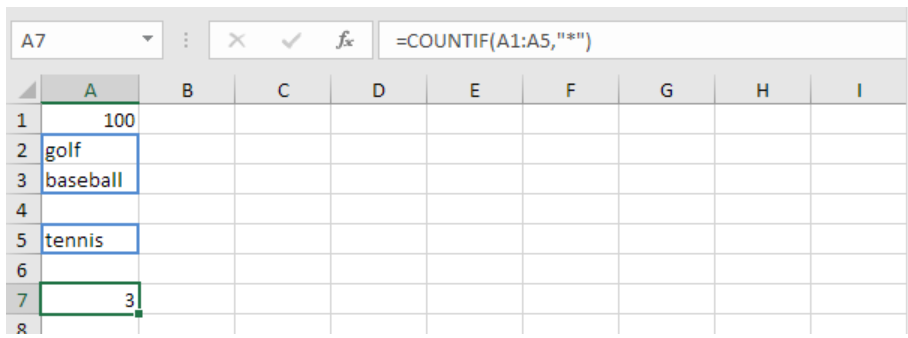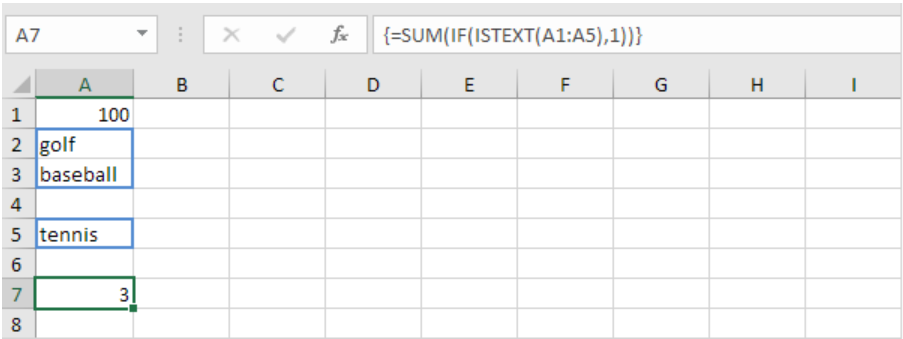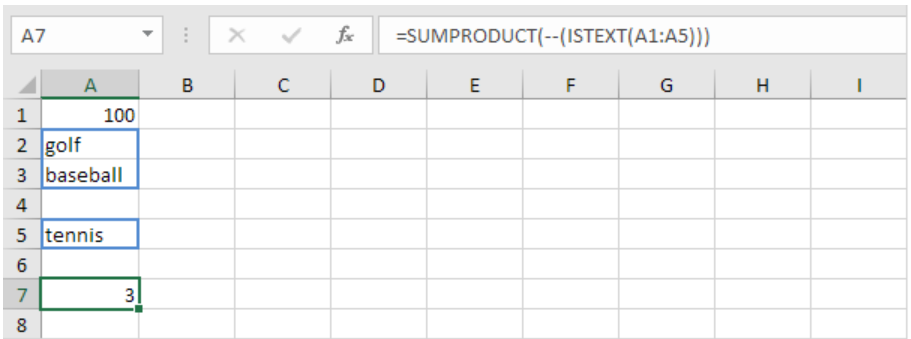Get instant live expert help with Excel or Google Sheets“My Excelchat expert helped me in less than 20 minutes, saving me what would have been 5 hours of work!”

#### Post your problem and you’ll get Expert help in seconds.

Your message must be at least 40 characters
Our professional Expert are available now. Your privacy is guaranteed.

# How to sum cells that contain text in Excel – Excelchat

As we work with Excel spreadsheets, we might be required to count all the cells that contain text. This would be a tedious work if we were to count it manually, especially when dealing with a large group of data. We can use the Excel sumif text criteria to get only those cells that have text in them, and not numbers. In this post, we shall explore various methods to find the sum of cells with text only.

## Using Excel formula if cell contains text then sum

Excel provides us with so many formulas do literally everything we can ever think of. To sum cells with text, we can use the SUMIF function to count the number of cells with text. The general formula shall look like the one below;

`=COUNTIF (rng, “*”)`

Where;

rng refers to the range of cells from which you want to count cells with text.

Notice that we have used the asterisk symbol (*) in the formula when counting text cells.Figure 1: Using COUNTIF function to count cells with text

## Using an array formula to get number of cells with text

We can also use an array formula to count cells with text. A combination of SUM, IF, IS and TEXT functions can help us know how many cells have text in a spreadsheet.

To get the number of cells with text in our above example, we shall use the formula below;

`{=SUM(IF(ISTEXT(rng), 1))}`

In the example in figure 1 above, we have the formula as;

`{=SUM(IF(ISTEXT(A1:A5), 1))}`Figure 2: Counting cells with text using an array formula

In figure 2 above, we have the formula;

`{=SUM(IF(ISTEXT(A1:A5),1))}`

When entering the above formula, press Ctrl + Shift + Enter.

## Using the SUMPRODUCT function to count number of cells with text

You can also use the SUMPRODUCT function to know the number of cells with text. To use the SUMPRODUCT function, select a cell and type in the following formula;

`=SUMPRODUCT(--(ISTEXT(rng)))`Figure 3: Using SUMPRODUCT to count cells with text

In figure 3 above, we have the formula below in cell A7;

`=SUMPRODUCT(--(ISTEXT(A1:A5)))`

When we press Enter, we shall have the total number of cells with text as 3 as shown in cell A7.

## Instant Connection to an Expert through our Excelchat Service

Most of the time, the problem you will need to solve will be more complex than a simple application of a formula or function. If you want to save hours of research and frustration, try our live Excelchat service! Our Excel Experts are available 24/7 to answer any Excel question you may have. We guarantee a connection within 30 seconds and a customized solution within 20 minutes.Guest
LAURIE GOLDSTEIN

How do i add up all the rows in a column that have any text in them?

Comment awaiting moderation